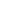# How To Use Name Numerology To Erect Hexagrams For Yi Jing

While the use of name numbers is mostly used for numerology, a person’s name can also be used to erect hexagrams dynamically to consult Yi Jing for divination purposes.

This is somewhat similar to how plum blossoms divination method are primarily used for.

To apply the name oracle method, we have to first convert a person’s into numbers.The following table is a reference to the 26 alphabets and the numbers they are tagged to.

 1 2 3 4 5 6 7 8 9 A B C D E F G H I J K L M N O P Q R S T U V W X Y Z

As a hexagram is made up of 2 trigrams, this method only requires 2 sets of numbers to determine trigrams.

As such, only the first and last name should be used. Leaving the middle name (if any).

With each letter in a name, the number tagged to it will be added to one another. The final number would then be divided by 8 as there are 8 trigrams.

For example, a name like Leonard would have a number of:

3+5+6+5+1+9+4 = 33

33/8 = ~4

So the trigram 4 of Zhen would make up the upper trigram in the hexagram. The lower trigram which makes up the remainder of the hexagram would be determined by the same method used for the last name.

To locate a changing line, we can add the numbers for the first and last name, with the Chinese hour of the event, then divide it by 5.

For example, if the even occurred at 6pm, it would be the hour of the Rooster which is the 10th 2-hour block according to Chinese time-keeping.

If the first trigram is 4 and the second trigram is 6, we can add them up by:

4+6+10 = 20

20/5 = 4

The reason why we use the number 5 for division is that the maximum number possible in this instance is 28. This is because there are only 8 trigrams, making the maximum possible number for the first and last name 8. And since there are 12 2-hour blocks in the Chinese day, the maximum number for the hour is 12.

When we add 8 to 8 to 12, we obtain the number 28.

And because there are 6 lines to each hexagram, the division number has to produce a final result of between 1 to 6.

5 is the only whole number capable of doing that to the number 28.

Most popular feng shui items on Amazon Come join the FB community here

This also means that you would use a different division number if you choose to use a time determined by the typical 24 hours.

For example, if you choose to use 18 (6pm), then the maximum total number would be 8+8+18 = 34. The division number would then be 6 since that is the only whole number capable of producing a final result of between 1 to 6.

Once the original hexagram and final hexagram is generated, refer to the I-Ching to interpret the meanings.

This name oracle method can be useful for seeking a quick divine answer to what the intentions of a person you’ve just met are. You would need a pocket copy of the Yi Jing with you.

The content provided on this website is free of charge. If you find the information useful, you can buy me a coffee here. And come join the FB community here
Get exclusive feng shui insights that you would not find anywhere else.
Submit

scroll to top
Go